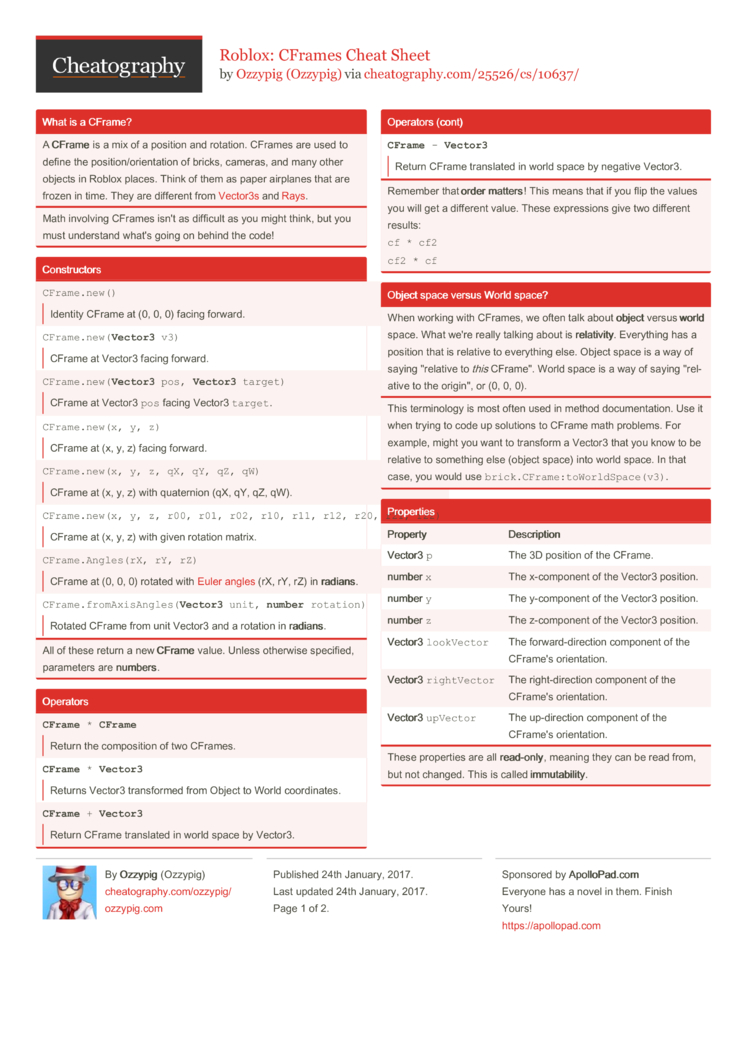# Roblox: CFrames Cheat Sheet by Ozzypig

A reference for using CFrames in Roblox Lua. Written for intermediate scripters.

### What is a CFrame?

 A CFrame is a mix of a position and rotation. CFrames are used to define the positi­on/­ori­ent­ation of bricks, cameras, and many other objects in Roblox places. Think of them as paper airplanes that are frozen in time. They are different from Vector3s and Rays.
Math involving CFrames isn't as difficult as you might think, but you must understand what's going on behind the code!

### Constr­uctors

 `CFrame.new()`Identity CFrame at (0, 0, 0) facing forward. `CFrame.new(Vector3 v3)`CFrame at Vector3 facing forward. `CFrame.new(Vector3 pos, Vector3 target)`CFrame at Vector3 `pos` facing Vector3 `target`. `CFrame.new(x, y, z)`CFrame at (x, y, z) facing forward. `CFrame.new(x, y, z, qX, qY, qZ, qW)`CFrame at (x, y, z) with quaternion (qX, qY, qZ, qW). `CFrame.new(x, y, z, r00, r01, r02, r10, r11, r12, r20, r21, r22)`CFrame at (x, y, z) with given rotation matrix. `CFrame.Angles(rX, rY, rZ)`CFrame at (0, 0, 0) rotated with Euler angles (rX, rY, rZ) in radi­ans. `CFrame.fromAxisAngles(Vector3 unit, number rotation)`Rotated CFrame from unit Vector3 and a rotation in radi­ans.
All of these return a new CFrame value. Unless otherwise specified, parameters are numb­ers.

### Operators

 `CFrame * CFrame`Return the compos­ition of two CFrames. `CFrame * Vector3`Returns Vector3 transf­ormed from Object to World coordi­nates. `CFrame + Vector3`Return CFrame translated in world space by Vector3. `CFrame - Vector3`Return CFrame translated in world space by negative Vector3.
Remember that order matters! This means that if you flip the values you will get a different value. These expressions give two different results:
`cf * cf2cf2 * cf`

### Object space versus World space?

 When working with CFrames, we often talk about object versus world space. What we're really talking about is rela­tiv­ity. Everything has a position that is relative to everything else. Object space is a way of saying "­rel­ative to this CFrame­". World space is a way of saying "­rel­ative to the origin­", or (0, 0, 0).
This terminology is most often used in method documentation. Use it when trying to code up solutions to CFrame math problems. For example, might you want to transform a Vector3 that you know to be relative to something else (object space) into world space. In that case, you would use `brick.CFrame:toWorldSpace(v3)`.

### Properties

 Prop­erty Desc­rip­tion Vector3 `p` The 3D position of the CFrame. number `x` The x-comp­onent of the Vector3 position. number `y` The y-comp­onent of the Vector3 position. number `z` The z-comp­onent of the Vector3 position. Vector3 `lookVector` The forwar­d-d­ire­ction component of the CFrame's orient­ation. Vector3 `rightVector` The right-­dir­ection component of the CFrame's orient­ation. Vector3 `upVector` The up-dir­ection component of the CFrame's orient­ation.
These properties are all read­-on­ly, meaning they can be read from, but not changed. This is called immu­tab­ili­ty.

### Methods

 CFrame `cf:inverse()`Returns the inverse of this CFrame. CFrame `cf:lerp(CFrame goal, number alpha)`Returns a CFrame interp­olated between this CFrame, and the goal CFrame by the fraction alpha. CFrame `cf:toWorldSpace(CFrame cf2)`Returns a CFrame transformed from Object to World coordinates. Equivalent to `cf * cf2` CFrame `cf:toObjectSpace(CFrame cf2)`Returns a CFrame transformed from World to Object coordinates. Equivalent to `cf:inverse() * cf2` Vector3 `cf:pointToWorldSpace(Vector3 v3)`Returns a Vector3 transformed from Object to World coordinates. Equivalent to `cf * v3` Vector3 `cf:pointToObjectSpace(Vector3 v3)`Returns a Vector3 transformed from World to Object coordinates. Equivalent to `cf:inverse() * v3` Vector3 `cf:vectorToWorldSpace(Vector3 v3)`Returns a Vector3 rotated from Object to World coordinates. Equivalent to `(cf - cf.p) * v3` Vector3 `cf:vectorToObjectSpace(Vector3 v3)`Returns a Vector3 rotated from World to Object coordinates. Equivalent to `(cf - cf.p):inverse() * v3` numbers `cf:components()`Returns the components of the CFrame in this order: x, y, z, R00, R01, R02, R10, R11, R12, R20, R21, R22. numbers `cf:toEulerAnglesXYZ()`Returns the best guess angles that could be used to generatea CFrame using `CFrame.Angles`. See Euler angles.

## Download the Roblox: CFrames Cheat Sheet2 Pages
//media.cheatography.com/storage/thumb/ozzypig_roblox-cframes.750.jpg

PDF (recommended)